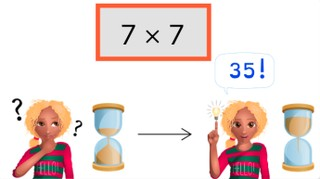Automaticity 7 times table

# Automaticity 7 times table

Students learn to memorize the 7 times table.8,000 schools use Gynzy92,000 teachers use Gynzy1,600,000 students use Gynzy

## General

The students learn to memorize the 7 times table.

## Standards

CCSS.Math.Content.3.OA.C.7

## Learning objective

Students will be able to memorize the 7 times table and quickly solve its products.

## Introduction

Drag the octopus through the maze to get to the water. The octopus can only take steps of 7 (0,7,14,21, etc). He can move right and left, and up and down. He just cannot take steps diagonally.

## Instruction

First have the students solve the 7 times table problems in 5, 4, and 3 seconds. Then show the pile of cards. Explain that you are going to start the timer and remove the cover to show the first problem. Have the students solve it quickly and then drag the card away to show the next problem. Try to solve all the problems within the time given by the timer.

Check whether the students have memorized the 7 times table with the following question:
- Why is it important to memorize the 7 times table?

## Quiz

The students practice their memorization of the 7 times table with 3 timed exercises.

## Closing

Discuss once again the importance of memorizing the 7 times table. To close the lesson, ask the students how quickly they think they can solve five problems from the 7 times table. Set the timer for that time, spin the wheel five times, and have the students solve the problems.

## Teaching tips

Give students that have difficulty memorizing the 7 times table a printed version of the 7 times table to practice with. Have them work in pairs with a timer to solve problems in shorter and shorter amounts of time.

## Instruction materials

You may print out a copy of the 7 times table.

### The online teaching platform for interactive whiteboards and displays in schools

• Save time building lessons

• Manage the classroom more efficiently

• Increase student engagement